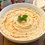# $\pi$!(2)

${ \pi }^{ 2 }=4+32\displaystyle\sum _{ n=1 }^{ \infty }{ \dfrac { n }{ (2n-1)(2n+1)^{ 2 } } }$

I was playing around with the expansion of $\ \dfrac { { x }^{ 2 } }{ \sqrt { 1-{ x }^{ 2 } } }$ this time, and I found the series above. Can you prove it?

For a related question, see this.Note by Hamza A
5 years, 5 months ago

This discussion board is a place to discuss our Daily Challenges and the math and science related to those challenges. Explanations are more than just a solution — they should explain the steps and thinking strategies that you used to obtain the solution. Comments should further the discussion of math and science.

When posting on Brilliant:

• Use the emojis to react to an explanation, whether you're congratulating a job well done , or just really confused .
• Ask specific questions about the challenge or the steps in somebody's explanation. Well-posed questions can add a lot to the discussion, but posting "I don't understand!" doesn't help anyone.
• Try to contribute something new to the discussion, whether it is an extension, generalization or other idea related to the challenge.

MarkdownAppears as
*italics* or _italics_ italics
**bold** or __bold__ bold
- bulleted- list
• bulleted
• list
1. numbered2. list
1. numbered
2. list
Note: you must add a full line of space before and after lists for them to show up correctly
paragraph 1paragraph 2

paragraph 1

paragraph 2

[example link](https://brilliant.org)example link
> This is a quote
This is a quote
    # I indented these lines
# 4 spaces, and now they show
# up as a code block.

print "hello world"
# I indented these lines
# 4 spaces, and now they show
# up as a code block.

print "hello world"
MathAppears as
Remember to wrap math in $$ ... $$ or $ ... $ to ensure proper formatting.
2 \times 3 $2 \times 3$
2^{34} $2^{34}$
a_{i-1} $a_{i-1}$
\frac{2}{3} $\frac{2}{3}$
\sqrt{2} $\sqrt{2}$
\sum_{i=1}^3 $\sum_{i=1}^3$
\sin \theta $\sin \theta$
\boxed{123} $\boxed{123}$

Sort by:

Using partial fractions, we can observe that: $\frac{32n}{(2n-1)(2n+1)^2} = \frac{4}{2n-1} - \frac{4}{2n+1} + \frac{8}{(2n+1)^2}$

Now $\sum_{n=1}^{\infty} \left(\frac{4}{2n-1} - \frac{4}{2n+1}\right)$ is a telescoping sum, whose value is $4$. So there is just one more sum to calculate: $\sum_{n=1}^{\infty} \frac{8}{(2n+1)^2} = 8\left(\sum_{k=1}^{\infty} \frac{1}{k^2} - \sum_{n=1}^{\infty} \frac{1}{(2n)^2} - \frac{1}{1^2}\right)$$= \frac{8\pi^2}{6} -\frac{8\pi^2}{4*6} - 8 = \pi^2 - 8$

Therefore, $4+32\sum_{n=1}^{\infty} \frac{n}{(2n-1)(2n+1)^2} = 4+(4+\pi^2-8) = \pi^2$QED

I'm curious how you got this from the series of $\frac{x^2}{\sqrt{1-x^2}}$?

- 5 years, 5 months ago

so ,i used the expansion of $\frac { { x }^{ 2 } }{ \sqrt { 1-{ x }^{ 2 } } }$ which is $\displaystyle\sum _{ n=1 }^{ \infty }{ \frac { \begin{pmatrix} 2n-2 \\ n-2 \end{pmatrix} }{ { 4 }^{ n-1 } } } { x }^{ 2n }$ integrated both sides then put $x=\sin{t}$ and i got

$\displaystyle\sum _{ n=1 }^{ \infty }{ \frac { \begin{pmatrix} 2n-2 \\ n-2 \end{pmatrix} }{ { 4 }^{ n-1 } } } \frac { { \sin^{2n+1}{t} } }{ 2n+1 } =\frac { t-\sin { t } \cos { t } }{ 2 }$

then i integrated from $0$ to $\frac{\pi}{2}$ and got with a lot of rearranging and simplifying and comparing with other sums i get ${ \pi }^{ 2 }=4+32\displaystyle\sum _{ n=1 }^{ \infty }{ \dfrac { n }{ (2n-1)(2n+1)^{ 2 } } }$

this is how it looked before all the comparing and substituting

$\frac { { \pi }^{ 2 } }{ 16 } -\frac { 1 }{ 4 } =\frac { 1 }{ 2 } \displaystyle\sum _{ n=1 }^{ \infty }{ \frac { \Gamma (n+1)\sqrt { \pi } \begin{pmatrix} 2n-2 \\ n-1 \end{pmatrix} }{ { 4 }^{ n-1 }(2n+1)\Gamma (n+\frac { 3 }{ 2 } ) } }$

this was a practice problem in my textbook,so i had faith in solving it ;),it was a bit tedious though

- 5 years, 5 months ago

Wow, looks like a lot of work! Nice job though!

- 5 years, 5 months ago

your method was way simpler,i should've used that one ;)

- 5 years, 5 months ago

You beated me while writing the solution.

- 5 years, 5 months ago

Two different Countries, two different Timezones but then also you two, writing the same solution of the same question at the same time. Wow! These is possible on Brilliant only. Cheers....

- 5 years, 5 months ago

Yeah !! You are right !!!! :P

- 5 years, 5 months ago

Interesting, we both had the same solution at the same time

- 5 years, 5 months ago

i'll post how once i get back from school :)

- 5 years, 5 months ago

$4+32\displaystyle\sum _{ n=1 }^{ \infty }{ \dfrac { n }{ (2n-1) (2n+1)^{ 2 } } } \\ 4+32 \displaystyle \sum^{\infty}_{n=1}\left( \frac{1}{8(2n-1)}-\frac{1}{8(2n+1)}+\frac{1}{4(2n+1)^2}\right) \\ 4+ \displaystyle \sum^{\infty}_{n=1} \left( \frac{4}{(2n-1)}-\frac{4}{(2n+1)}+\frac{8}{(2n+1)^2}\right) \\ 4+\left( 4+\frac{4}{3}+\frac{4}{5}+\ldots -\frac{4}{3}-\frac{4}{5}-\ldots +8\underbrace\sum^{\infty}_{n=1} \frac{1}{(2n+1)^2}}_\sum^{\infty}_{n=1}\frac{1}{n^2}-\displaystyle \sum^{\infty}_{n=1}\frac{1}{(2n)^2}-1 = \frac{\pi^2}{6}-\frac{\pi^2}{24}-1=\frac{\pi^2}{8}-1 } \right) \\ 8+8\left(\frac{\pi^2}{8}-1\right) \\ 8+\pi^2-8=\boxed{\pi^2$

- 5 years, 5 months ago

Very neat solution! +1

- 5 years, 4 months ago

Thank you very much ! :-)

- 5 years, 4 months ago